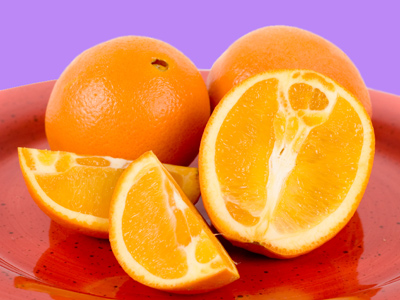24 is the same as 12 and 44 is the same as one whole.

# Equivalent Fractions

This Math quiz is called 'Equivalent Fractions' and it has been written by teachers to help you if you are studying the subject at elementary school. Playing educational quizzes is an enjoyable way to learn if you are in the 3rd, 4th or 5th grade - aged 8 to 11.

It costs only \$12.50 per month to play this quiz and over 3,500 others that help you with your school work. You can subscribe on the page at Join Us

Recognising, writing and naming fractions are all important steps. Equivalent fractions means two or more fractions which have the same value. For example, two quarters and one half are both the same, as are two halves, four quarters and 1 whole.

It is important for children to be able to recognize equivalent fractions and this quiz will help them to do so.

Use this quiz to see how well you do with equivalent fractions.

1.
Kim ate 14 of a pizza and Fran also ate 14. What fraction of the pizza remains?
14
34
13
12
There are four quarters in a whole. If two are eaten, two remain and this is the same as a half
2.
Jim and Harry both have 12 jelly beans. Jim eats 14 of his and Harry eats 13 of his. Who ate more jelly beans?
Jim
Harry
They ate the same
Neither
14 of 12 is 3 and 13 of 12 is 4, so Harry ate one more than Jim
3.
Which fraction is the same as 12?
34
14
25
24
There are four quarters in a whole, so two of them would be the same as a half
4.
Which is less: 14 of 12 or 13 of 9?
They both give the same answer
13 of 9
14 of 12
13 of 12
13 of 9 is 3, and 14 of 12 is also 3
5.
If I cut a cake into four equal slices and eat two of them, how much have I eaten?
A third of the cake
A quarter of the cake
Half of the cake
Three quarters of the cake
Two quarters out of four is the same as one half
6.
Which is more: 13 of 12 or 12 of 12?
They are the same
13 of 12
14 of 12
12 of 12
12 of 12 is 6 and 13 of 12 is 4
7.
Which is less: 14 of 12 or 34 of 16?
34 of 16
24 of 16
14 of 12
14 of 16
34 of 16 is 12, 14 of 12 is only 3
8.
Jane is given \$24 for her birthday. She spends half the money on toys, a quarter of the money on candies and gives a quarter to charity. How much money does Jane have left?
\$2
\$0
\$1
\$4
A half added to a quarter and another quarter equals everything!
9.
Which is more: 12 of 6 or 34 of 8?
34 of 8
12 of 6
They are the same
13 of 6
34 of 8 is 6, and 12 of 6 is 3
10.
Which pair of fractions have the same value?
23 and 12
14 and 24
44 and 22
13 and 24
Whenever the numerator and denominator are the same number, the fraction is equal to one whole
Author:  Angela Smith